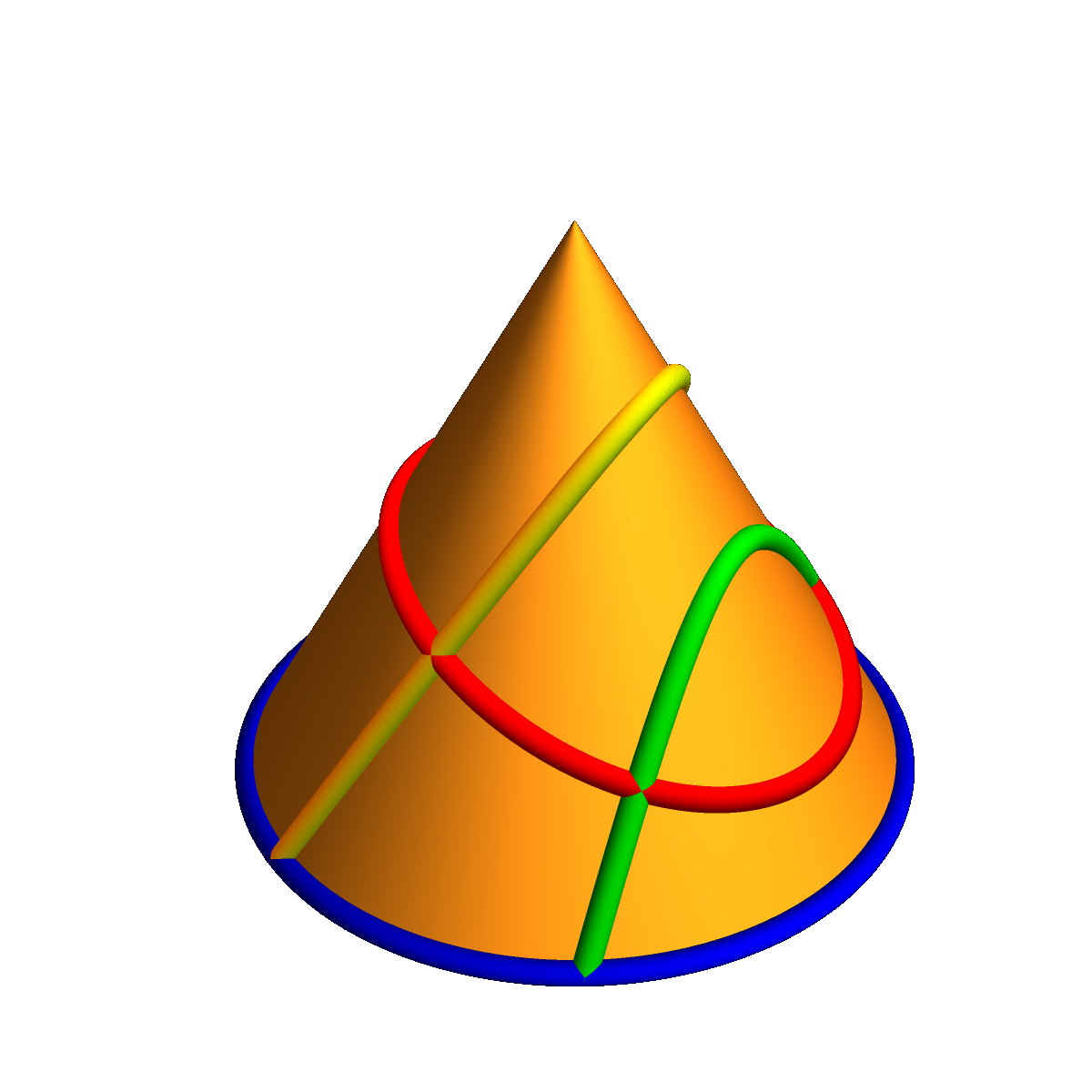When entering the course, you already knew the conic sections parabola, ellipse and hyperbola. You have learned now about quadric surfaces like paraboloids, ellipsoids and hyperboloids including limiting cases like cones or cylinders. You have also learned to parametrize all these surfaces. The ellipsoid using spherical coordinates, the hyperboloid using surfaces of revolutions and the paraboloids as graphs. Most of these parametrizations involve trig functions. One can now wonder, whether it is possible to do without them. That leads to the question of rational parametrizations. Remember that a rational function is a function of the form p(x)/q(x), where p,q are both polynomials. Finding rational parametrizations of curves or surfaces is a topic which belongs to the field of algebraic geometry. In general, given a rational surface, it can be tricky or even be impossible to find a rational parametrization. For conic sections or quadrics, it is possible however to do everything using rational functions.## Rationally parametrized curves

Lets look at conic sections, the parabola, ellipse and hyperbola. Parabola are already rationally parametrized by
```r(t) = < t, t2 >.
```
What about the circle? The common parametrizations r(t) = < cos(t),sin(t) > or r(t) = < t,(1-t2)1/2 > (for the upper half circle) are both not rational, as they involve trigonometric functions or square roots. There is a parametrization although which is rational: it is
```   r(t) = < 2t/(1+t^2), (1-t^2)/(1+t^2) >
```
It is rational because every of the entries x(t) and y(t) are rational functions. Now something for you: how can you modify this to parametrize the ellipse x2/a2 +y2/b2 = 1? Not every curve given by rational functions in x, y can be parametrized by rational functions. There are curves like y2 = x3 + x + 1 which can not be rationally parametrized. This is not so obvious, but you can read more about such here. The hyperbola could be parametrized as
```   r(t) = < (1+t2)/(2t),(t^2-1)/(2t) > .
```
And again, you can easily modify this to parametrize a more general hyperbola like x2/a2 -y2/b2 = 1.

## Rationally parametrized surfaces

Now, for quadrics, both the elliptic and hyperbolic paraboloid are easy to parametrize in a rational way as they are already given as such:
```r(u,v) = < u,v,u2+v2 >
```
for example is the parametrization of the elliptic paraboloid z=x2 + y2.
To parametrize the hyperboloid with rational functions, one has to be more creative. It is actually possible even to find a rational parametrization for which the grid curves are straight lines. The following form has been shown to me by Jun-Hou Fung:
```r(u,v) = < (u + v)/(u - v),
(u v - 1)/(u - v),
(u v + 1)/(u - v) >
```
who provided also a short Mathematica proof of that fact and a verification that the grid curves are straight lines: the computation shows that the grid curves have zero curvature:
```r[u_, v_] := {(u + v)/(u - v), (u v - 1)/(u - v), (u v + 1)/(u - v)}
r[u, v][]^2 + r[u, v][]^2 - r[u, v][]^2 == 1 // Simplify
Cross[D[r[u, b], u], D[r[u, b], {u, 2}]] == {0, 0, 0}
Cross[D[r[a, v], v], D[r[a, v], {v, 2}]] == {0, 0, 0}
```
If you want to know how Jun-Hou got this, talk to him. He got it from a so called Segre embedding which is way beyond what we do in this course.
An other (related by a change of variable) example is given in this stack exchange: it is
```r(u,v) = < (u v+1)/(v u-1),
(u-v)/(u v-1),
(u+v)/(u v-1) >
```
for which we can also run the code of Jun Hou and so answering that stack exchange question:
```r[u_, v_] := {(u v+1)/(v u-1), (u-v)/(u v-1), (u+v)/(u v-1) }
r[u, v][]^2 + r[u, v][]^2 - r[u, v][]^2 == 1 // Simplify
Cross[D[r[u, b], u], D[r[u, b], {u, 2}]] == {0, 0, 0}
Cross[D[r[a, v], v], D[r[a, v], {v, 2}]] == {0, 0, 0}
```
Here is a rational parametrization of the sphere (given for example in this lecture):
```r(u,v) = <
[(1 - u2)(1 - v2)]/[(1 + u2)(1 + v2)]
[-2 u (1 - v2)]/[(1 + u2)(1 + v2)]
2v /(1 + v2) >
```
Again, we could do the verification that x2 + y2 + z2 = 1 by hand but its much more gentle to just run it through the computer algebra system
```r[u_, v_] := {(1-u^2) (1-v^2)/((1+u^2)(1+v^2)),-2u(1-v^2)/((1+u^2)(1+v^2)),2v/(1+v^2)}
r[u, v][]^2 + r[u, v][]^2 + r[u, v][]^2 == 1 // Simplify
```
Again, how would you modify this to parametrize an arbitrary ellipsoid?
Now, this is pretty cool, but why do we still do the parametrization using trig functions? The answer is that even in the sphere case, we would need the entire plane u,v even to cover the sphere and that there are parts then which are covered multiple times. Look at the picture:
```r[u_, v_] := {(1-u^2) (1-v^2)/((1+u^2)(1+v^2)),-2u(1-v^2)/((1+u^2)(1+v^2)),2v/(1+v^2)}
ParametricPlot3D[r[u, v], {u, -6, 6}, {v, -6, 6}]
```
In the hyperboloid example, the picture is a mess as there are places where we divide by zero.